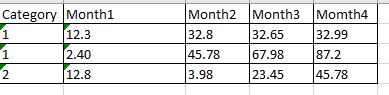# Find 1 decimal place values in datatable

Hi,
I have a datatable, somewhat like the one shown in snip belowI need to find out all 1 decimal value numbers and append 0 to it thus making it 2 decimal place. e.g. in above - 32.8 changes to 32.80
Please can someone help with most optimized way, I thought of iterating through the Dt and passing each item through a regex for 1 decimal place “^\d+(.\d{1})\$”, if match found then simply append 0 to it.
This might not be an efficient way to do, please can someone help me, if there is some LINQ for it.

@Faraz_Subhani,
I assume DataType for each such column is Double. So while displaying, you can use variableName.ToString(“0.00”). It will convert all numeric value with 2 decimal point. e.g. 12.3 will be converted to 12.30.

Please let me know if that is not the case.

1. decimalVar.ToString(“F”)
2. decimalVar.ToString(“0.00”)
3. decimalVar = decimal.Round(decimalVar, 2, MidpointRounding.AwayFromZero);

Thanks for quick response, but I need to convert to 2 decimal place only if 1 decimal place value is found. Whole numbers should be left as it is but if it has got 1 decimal place e.g 32.5 final value should be 32.50

@Faraz_Subhani If you take an Example of 32.5 , take this value as String, Then Split it Based on “.” then take the 2nd value and Compare it’s Length, If it’s 1 Then Use the Methods suggested above else do not do anything.
I hope you can understand thisTo get the Splitted Value and compare it with 1, you can use this:
Split(“32.5”,“.”)(1).Trim.Count>1

@Faraz_Subhani , This is exactly how it will work. Have you tried?

Yes Bro I tried, so for instance if there is whole number the output coming is 60.00 but ai need to convert to 2 decimal values only 1 decimal value found, whole numbers should remain as it is

Approach looks correct but again it will become quite lengthy and inefficient as Was the case with my approach because we will have to iterate through datatable and pass each cell value in regex and check for 1 decimal place if exists then append 0

@Faraz_Subhani How many Columns are present ?

That is being created dynamically bro based on prior values extracted

I tried your solution but again it alters whole numbers as well, I want the whole numbers to remain as it is

Have you tried all my methods?

None of the methods seems to be helpful?It works properly as said for decimal values but it it changes whole numbers as well for eg 60 becomes 60.00 but I want whole numbers to remain as it is.
Any other suggestion, else I think I have to go the harder way to pass each and every cell value through regex and check if it has decimal only then convert to 2 decimal values

@Palaniyappan please can you throw some light on this

1 Like

You can check whether it has a decimal or not and then you can use the above methods and solve

Like

If condition string.contains(“.”)

If your excel has the column as decimal, then i dont think you can have one cell as 60 and another as 59.11, all need to have the same format (60.00), for this, most likely you will need to save the values as text…

1 Like

As the above methods were not working exactly, so I tried to iterate through entire datatable and check for each cell value using pattern matching, if value contains one decimal place then append ‘0’ to it thus making it a 2 decimal value( I cannot round of the values).
It works fine, but the only problem is that, when assigning back the appended value to datatable it truncates 0 again making it again a 1 decimal value.

I think it is something related to datatype which I am using, attaching the test workflow, please if someone can help on this.

I understand that while writing it back to excel it will truncate the value, but while reading it simply from datatable it should have the value appended with 0.

ConsolidateTableTest.zip (22.8 KB)